Home > A2C > Chapter 11 > Lesson 11.3.3 > Problem11-161

11-161.
1. Solve and graph each inequality. Homework Help ✎

1.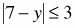2.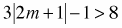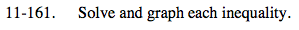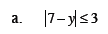Solve this inequality like a normal equation, just use the less than symbol as the equals sign.

Remember to solve both 7 − y ≤ 3 and 7 − y ≥ −3.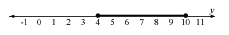4 ≤ y ≤ 10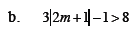Use the same method as part (a).